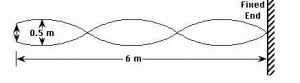NEET  >  Test: Standing Waves

# Test: Standing Waves

Test Description

## 5 Questions MCQ Test Physics Class 11 | Test: Standing Waves

Test: Standing Waves for NEET 2023 is part of Physics Class 11 preparation. The Test: Standing Waves questions and answers have been prepared according to the NEET exam syllabus.The Test: Standing Waves MCQs are made for NEET 2023 Exam. Find important definitions, questions, notes, meanings, examples, exercises, MCQs and online tests for Test: Standing Waves below.
Solutions of Test: Standing Waves questions in English are available as part of our Physics Class 11 for NEET & Test: Standing Waves solutions in Hindi for Physics Class 11 course. Download more important topics, notes, lectures and mock test series for NEET Exam by signing up for free. Attempt Test: Standing Waves | 5 questions in 10 minutes | Mock test for NEET preparation | Free important questions MCQ to study Physics Class 11 for NEET Exam | Download free PDF with solutions
 1 Crore+ students have signed up on EduRev. Have you?
Test: Standing Waves - Question 1

### A node is a point where there is always

Detailed Solution for Test: Standing Waves - Question 1

A node is a point along the medium of no displacement. The point is not displaced because destructive interference occurs at this point.

Test: Standing Waves - Question 2

### A cylindrical tube, open at both ends, has a fundamental frequency f in air. The tube is dipped vertically in water so that half of it is in water. The fundamental frequency of the air-column is now:

Detailed Solution for Test: Standing Waves - Question 2

As we know, f=v/2l
Now, it will act as one end and one end closed.
So, f0=v/2l’=v/4½=v/2l=f

Test: Standing Waves - Question 3

### Fundamental note in open pipe (v1 = ν/2L) has _________ the frequency of the fundamental note in closed organ pipe (v2 = ν/4L).

Detailed Solution for Test: Standing Waves - Question 3

Let L be a length of the pipe,
The fundamental frequency of closed pipe is
v2​=ν​/4L                                         .....(i)
where ν is the speed of sound in air.
Fundamental frequency of open pipe of same length is
v​1=ν/2L​                                            .....(ii)
After dividing v1 with v2,
v1/v2= ν/2L/ ν​/4L
v1=2v2

Test: Standing Waves - Question 4

Equation of longitudinal stationary waves in the pipe is

Test: Standing Waves - Question 5

In an experiment to find the speed of waves in a rope, a standing wave pattern is established as shown in diagram below. The vibrating end of rope makes 90 vibrations per minute. The speed of the waves isDetailed Solution for Test: Standing Waves - Question 5

If there are exactly 90 vibrations in 60.0 seconds, then there is a frequency of 1.5 Hz. The diagram shows 1.5 waves in 6.0-meters of rope; thus, the wavelength is 4.0 meters. Now use the equation v=f*w to calculate the speed of the wave. Proper substitution yields 6.0 m/s.

## Physics Class 11

127 videos|464 docs|210 tests
 Use Code STAYHOME200 and get INR 200 additional OFF Use Coupon Code
Information about Test: Standing Waves Page
In this test you can find the Exam questions for Test: Standing Waves solved & explained in the simplest way possible. Besides giving Questions and answers for Test: Standing Waves, EduRev gives you an ample number of Online tests for practice

## Physics Class 11

127 videos|464 docs|210 tests

### How to Prepare for NEET

Read our guide to prepare for NEET which is created by Toppers & the best Teachers